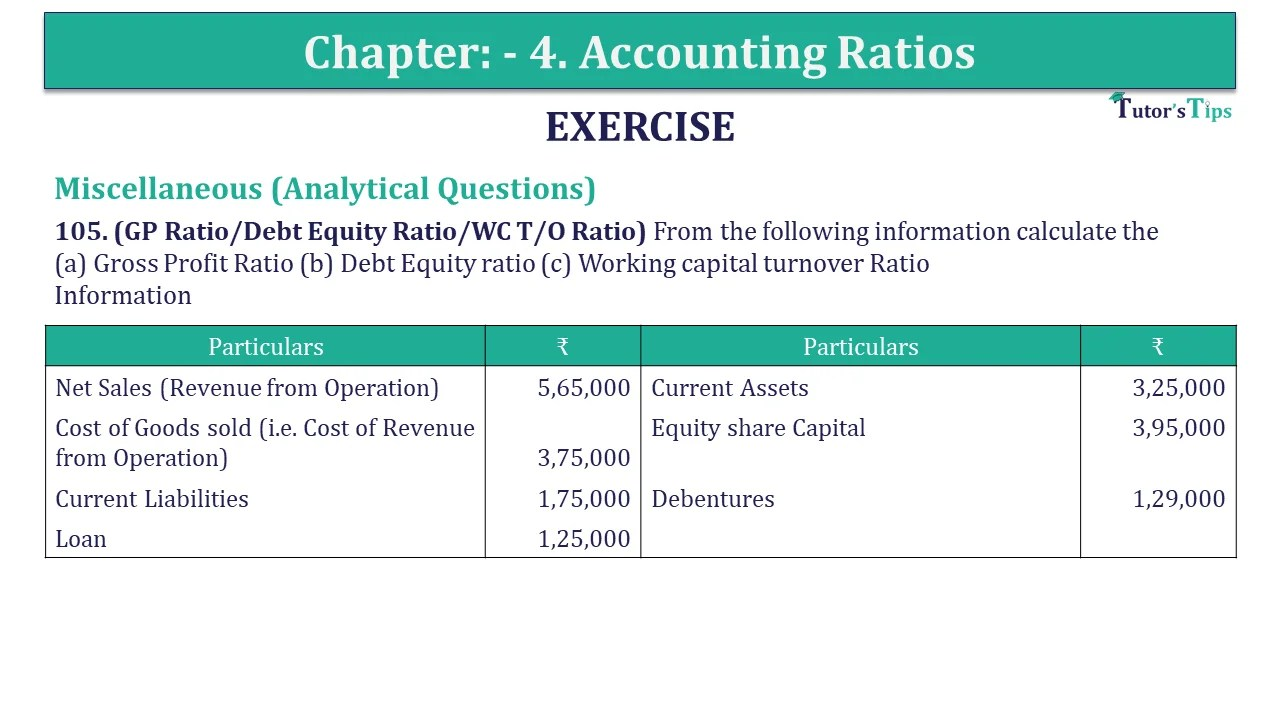# Question 105 Chapter 4 of +2-B – USHA Publication 12 ClassQ-105- CH-4 Book 2 - Usha Pub. +2 Book 2020 - Solution

Question 105 Chapter 4 of +2-B

Miscellaneous (Analytical Questions)

105. (GP Ratio/Debt Equity Ratio/WC T/O Ratio) From the following information calculate the
(a) Gross Profit Ratio (b) Debt Equity ratio (c) Working capital turnover Ratio
Information

 Particulars ₹ Particulars ₹ Net Sales (Revenue from Operation) 5,65,000 Current Assets 3,25,000 Cost of Goods sold (i.e. Cost of Revenue from Operation) 3,75,000 Equity share Capital 3,95,000 Current Liabilities 1,75,000 Debentures 1,29,000 Loan 1,25,000

## The solution of Question 105 Chapter 4 of +2-B: –

 (i) Gross Profit Ratio = Gross Profit X 100 Net Sales = ₹ 1,90,000 X 100 ₹ 5,65,000 = 33.62%

 (ii) Debt Equity Ratio = Debt X 100 Equity = ₹ 2,54,000 X 100 ₹ 3,95,000 = 0.64 : 1

 (iii) Working Capital Turnover Ratio = Sales Working Capital = ₹ 5,65,000 ₹ 1,50,000 = 3.76 times

 Gross Profit = Sales – Cost of goods sold = ₹ 5,65,000 – ₹ 3,75,000 = ₹ 1,90,000 Debt = Debentures + Loan = ₹ 1,29,000 + ₹ 1,25,000 = ₹ 2,54,000 Working Capital = Current Assets – Current Liabilities = ₹ 3,25,000 – ₹ 1,75,000 = ₹ 1,50,000

Also, Check out the solved question of previous Chapters: –